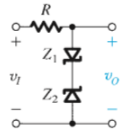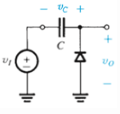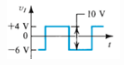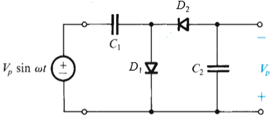Courses

# Test: Limiting And Clamping Circuits And Some Special Types Of Diodes

## 10 Questions MCQ Test Electronic Devices | Test: Limiting And Clamping Circuits And Some Special Types Of Diodes

Description
This mock test of Test: Limiting And Clamping Circuits And Some Special Types Of Diodes for Electrical Engineering (EE) helps you for every Electrical Engineering (EE) entrance exam. This contains 10 Multiple Choice Questions for Electrical Engineering (EE) Test: Limiting And Clamping Circuits And Some Special Types Of Diodes (mcq) to study with solutions a complete question bank. The solved questions answers in this Test: Limiting And Clamping Circuits And Some Special Types Of Diodes quiz give you a good mix of easy questions and tough questions. Electrical Engineering (EE) students definitely take this Test: Limiting And Clamping Circuits And Some Special Types Of Diodes exercise for a better result in the exam. You can find other Test: Limiting And Clamping Circuits And Some Special Types Of Diodes extra questions, long questions & short questions for Electrical Engineering (EE) on EduRev as well by searching above.
QUESTION: 1

### Which of the following circuits can be used as limiter or clamper or both?

Solution:

Each of the circuit can be either as a clamper or limiter or both.

QUESTION: 2

### The V0 vs VI curve for the below circuit isSolution:

Only this curve represents the characteristics correctly.

QUESTION: 3

### For the circuit and the input signal shown which of the following is true for the output voltage?Solution:

It is a clamped capacitor circuit.

QUESTION: 4

Which of the following is not true for the duty cycle of a waveform?

Solution:

60% duty time means that a signal is active for 60% of the total time.

QUESTION: 5

The maximum and the minimum voltage across the diode D1 respectively is​Solution:

It is a voltage doubler circuit.

QUESTION: 6

Limiting and clamping circuits are employed in

Solution:

All of the given are uses of limiting and clamping circuits.

QUESTION: 7

Which of the following is not true for a Schottky-Barrier Diode (SBD)?

Solution:

Current is conducted largely by electrons which are majority current carriers.

QUESTION: 8

Photodiodes are part of

Solution:

Optoelectronics are also called photodiodes.

QUESTION: 9

Which of the following is true for LEDs

Solution:

The working of the optoelectronics is opposite to that of the LEDs.

QUESTION: 10

Optoisolator is

Solution:

By definition, an optoisolator is a package containing both LED as well as a photodiode.